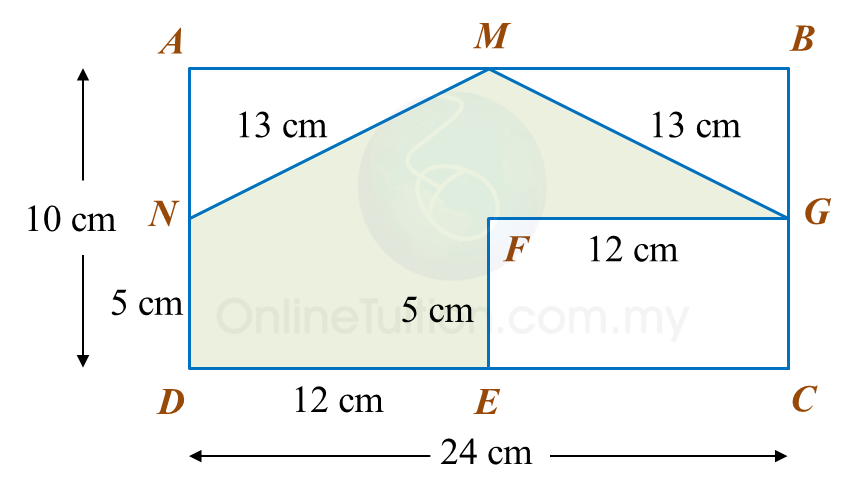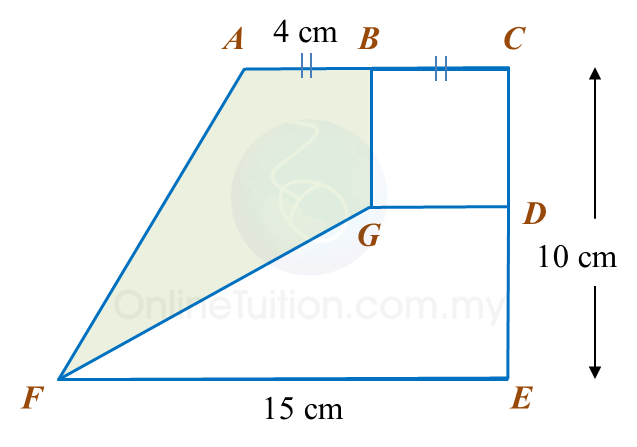# 11.2.2 Perimeter and Area, PT3 Practice

 
                   
Question 5:
 
In diagram below, PQUV is a square, QRTU is a rectangle and RST is an equilateral triangle.The perimeter of the whole diagram is 310 cm.
 Calculate the length, in cm, of PV.
 
 Solution:
                               
 
 
 
 
                   
Question 6:
 
In diagram below, ABCD and CGFE are rectangles. M, G, E and N are midpoints of AB, BC, CD and DA respectively.Calculate the perimeter, in cm, of the coloured region.
 
 Solution:Question 7:
 Diagram below shows a trapezium BCDE and a parallelogram ABEF. ABC and FED are straight lines.The area of ABEF is 72 cm2.
 Calculate the area, in cm2, of trapezium BCDE.
 
 Solution:
        

 
 
 
     
Question 8:
 In diagram below, ACEF is a trapezium and BCDG is a square.Calculate the area, in cm2, of the coloured region.
 
 Solution: